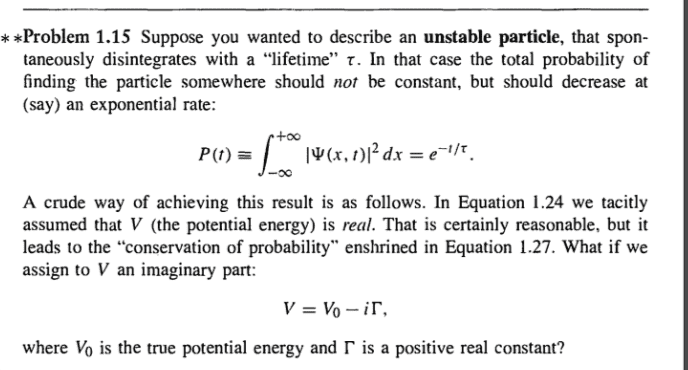# What does complex potential energy mean for a particle?

• I
• pintu935
In summary, the conversation discusses the concept of imaginary potential energy and its use in numerical calculations, particularly in quantum systems. The idea is a computational trick and can be used to calculate the ground state of a system. There is also mention of the Wigner-Weisskopf approximation, which is used to understand decay, and a link to a clear derivation on the topic.

#### pintu935

Griffith says in problem 1.15 the potential energy has an imaginary part. my question is that any real case exists where the part of the potential energy is imaginary?#### Attachments

Measurable physical quantities like energy can never be complex numbers. The thing described there is just a computational "trick". A similar effect can be produced by setting a complex mass or time variable. This can be used in the numerical calculation of the ground state of a quantum system, as I have done in this blog post of mine: https://physicscomputingblog.com/20...art-5-schrodinger-equation-in-imaginary-time/

•pintu935

Sigh :-(. Is this again from Griffiths's QM textbook? I cannot understand how an expert of the subject can be so sloppy and confusing for students. That's the more ununderstandable since he's obviously a brillant teacher of physics, as one can get from reading is electromagnetics textbook and many articles in AJP.

To understand "decay", particularly the exponential-decay law (which is necessarily an approximation only), you need to consider time-dependent perturbation theory. The standard treatment is known as Wigner-Weisskopf approximation. Here's a very clear derivation on the example of spontaneous emission, but it's of course applicable very generally

https://www.mpi-hd.mpg.de/personalhomes/palffy/Files/Spontaneous.pdf

•pintu935
vanhees71 said:
Sigh :-(. Is this again from Griffiths's QM textbook? I cannot understand how an expert of the subject can be so sloppy and confusing for students. That's the more ununderstandable since he's obviously a brillant teacher of physics, as one can get from reading is electromagnetics textbook and many articles in AJP.

To understand "decay", particularly the exponential-decay law (which is necessarily an approximation only), you need to consider time-dependent perturbation theory. The standard treatment is known as Wigner-Weisskopf approximation. Here's a very clear derivation on the example of spontaneous emission, but it's of course applicable very generally

https://www.mpi-hd.mpg.de/personalhomes/palffy/Files/Spontaneous.pdf
Thank you.